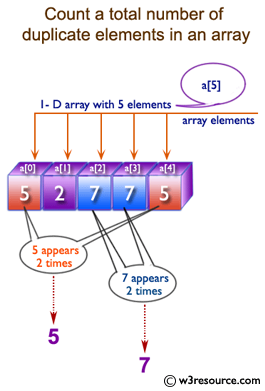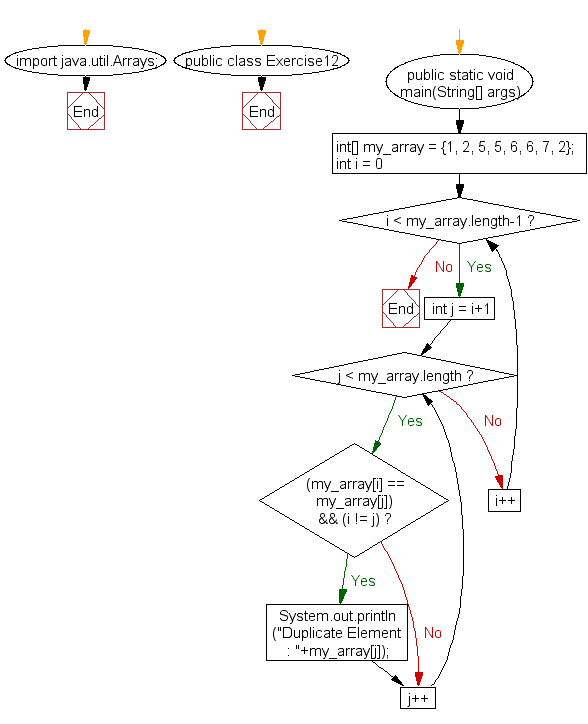﻿ Java: Find the duplicate values of an array of integers# Java Array Exercises: Find the duplicate values of an array of integer values

## Java Array: Exercise-12 with Solution

Write a Java program to find the duplicate values of an array of integer values.

Pictorial Presentation:Sample Solution:

Java Code:

``````import java.util.Arrays;
public class Exercise12 {
public static void main(String[] args)
{
int[] my_array = {1, 2, 5, 5, 6, 6, 7, 2};

for (int i = 0; i < my_array.length-1; i++)
{
for (int j = i+1; j < my_array.length; j++)
{
if ((my_array[i] == my_array[j]) && (i != j))
{
System.out.println("Duplicate Element : "+my_array[j]);
}
}
}
}
}
```
```

Sample Output:

```Duplicate Element : 2
Duplicate Element : 5
Duplicate Element : 6
```

Flowchart:Visualize Java code execution (Python Tutor):

Java Code Editor:

Improve this sample solution and post your code through Disqus

What is the difficulty level of this exercise?

Test your Programming skills with w3resource's quiz.

﻿

## Java: Tips of the Day

countOccurrences

Counts the occurrences of a value in an array.

Use Arrays.stream().filter().count() to count total number of values that equals the specified value.

```public static long countOccurrences(int[] numbers, int value) {
return Arrays.stream(numbers)
.filter(number -> number == value)
.count();
}
```

Ref: https://bit.ly/3kCAgLb